Display Accessibility Tools

##6-1 Prime Time

In Prime Time, students will explore important properties of whole numbers. Many of these properties are related to multiplication and division. The Investigations will help students understand relationships among factors, multiples, divisors, and products. Students will also learn how the Distributive Property relates multiplication and addition. The Investigations in this Unit will help students understand the following ideas.

• Classify numbers as prime or composite
• Recognize which situations call for common factors, common multiples, the least common multiple, or the greatest common factor
• Develop strategies for finding factors and multiples, least common multiples, and greatest common factors
• Recognize and use the fact that every whole number can be written in exactly one way as a product of prime numbers
• Use exponential notation to write repeated factors
• Relate the prime factorization of two numbers to the least common multiple and greatest common factor of two numbers
• Recognize that the Distributive Property relates the multiplicative and additive structures of whole numbers
• Use the properties of operations of numbers, including the Distributive Property and the Order of Operations convention, to write equivalent numerical expressions
• Use factors and multiples to solve problems, explain some numerical facts of everyday life

When your child encounters a new problem, it is a good idea to ask these questions. In this Unit, you might ask questions such as:

• Will breaking a number into factors help me solve the problem?
• What common factors and common multiples do the numbers have?
• What do the factors and multiples of the numbers tell me about the situation?
• When might it be useful to write a number in factored form or as a sum?

##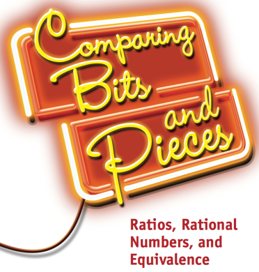6-2 Comparing Bits and Pieces

In Comparing Bits and Pieces, your child will develop skills in using fractions, decimals, ratios and percents to measure and to compare quantities.

• Use ratio language and notation to compare quantities
• Distinguish between fractions as numbers and ratios as comparisons
• Use a variety of scaling and partitioning strategies to reason proportionally
• Think of fractions and decimals as both locations and distances on the number line
• Move flexibly among fraction, decimal, and percent representations
• Find absolute values and opposites, and use them to describe real world quantities
• Use fraction, decimal, and percent benchmarks to estimate numbers
• Use context, models, drawings, or estimation to reason about situations
• Use equivalence of fractions and ratios to solve problems
• Use rate tables and unit rates to solve problems

As your child works on the problems in this Unit, ask yourself questions about situations that involve fractions, decimals, ratios and percents.

• What models or diagrams might be helpful in understanding the situation and the relationships among quantities?
• Is this a comparison situation? If so, do I use ratios or subtraction?
• What strategies can I use to find equivalent forms of these fractions, decimals, ratios, or percents?
• What strategies can I use to compare or order a set of fractions, decimals, and percents?
• What strategies can I use to reason about numbers greater than or less than 0?
• How can I use unit rates or rate table to make comparisons?

##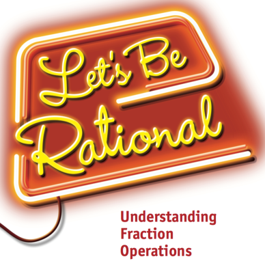6-3 Let's Be Rational

In Let’s Be Rational, your student will develop an understanding of the four basic arithmetic operations with fractions, including mixed numbers. They will also describe strategies for using these operations when solving problems involving fractions.

Your child will learn how to:

• Use benchmarks and other strategies to make reasonable estimates for results of operations with fractions, including mixed numbers
• Develop ways to model sums, differences, products, and quotients, including the use of areas, fraction strips, and number lines
• Look for rules to generalize patterns in fraction operations
• Use your knowledge of fractions, equivalence of fractions, and properties of numbers to develop algorithms for adding, subtracting, multiplying, and dividing fractions
• Recognize when addition, subtraction, multiplication, or division is the appropriate operation to solve a problem
• Write fact families to show the inverse relationship between addition and subtraction, and between multiplication and division
• Solve problems using operations on fractions, including mixed numbers
• Find values for variables by using operations on fractions, including mixed numbers

When your child encounters a new problem, it is a good idea to ask them questions such as:

• What models or diagrams might be helpful in understanding the problem situation and the relationships among quantities?
• What models or diagrams might help you decide which operation is useful in solving a problem?
• What is a reasonable estimate for the answer?

##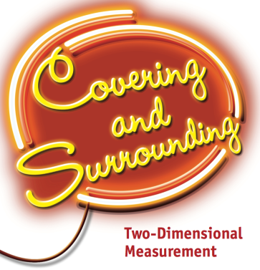6-4 Covering and Surrounding

In Covering and Surrounding, your student will explore areas and perimeters of figures. Attention is given especially to quadrilaterals and triangles. Your child will also explore surface area and volume of rectangular prisms. The Investigations in this Unit will help them

• Analyze what it means to measure area and perimeter
• Relate perimeter to surrounding a figure and area to covering a figure
• Develop strategies, procedures, and formulas, stated in words or symbols, for finding areas and perimeters of rectangles, parallelograms, and triangles
• Investigate relationships between perimeter and area, including that one can vary while the other stays fixed
• Analyze how the area of a triangle and the area of a parallelogram are related to the area of a rectangle
• Use nets that are made from rectangles and triangles to find surface areas of prisms
• Find the volume of rectangular prisms with fractional side lengths
• Use perimeter, area, surface area, and volume to solve problems.

When your child encounters a new problem, it is a good idea to ask questions such as:

• What attributes of a shape are important to measure?
• Is an exact answer required?
• How do I recognize whether area or perimeter of a figure is involved?
• What am I looking for when I find area? When I find perimeter?
• What relationships involving area, perimeter, or both, will help solve the problem?
• How can I determine the surface area of a prism from a net or a three-dimensional representation of the prism?
• What is the difference between area of a two-dimensional figure and surface area of a prism?

##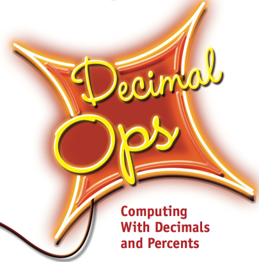6-5 Decimal Ops

In Decimal Ops, your student will learn how to make sense of and use the four operations (+, -, x, ÷,) on decimal numbers. Your child will also improve your understanding of and skill in working with percents.

Your student will learn how to:

• Add, subtract, multiply, and divide decimals
• Estimate the results of decimal operations
• Know when to use each operation in a situation involving decimals
• Relate operations on decimals to problems involving unit rates
• Use percents to solve problems

When your child encounters a new problem, it is a good idea to ask questions such as:

• Which operations on decimals or percents will help in solving this problem?
• What algorithms will help with the calculations?
• About how much will the sum, difference, product, or quotient be?
• What do the decimals and/or percents in the problem tell me about the situation?

##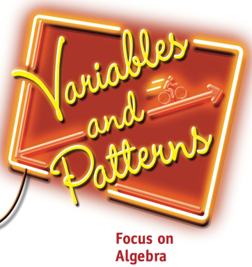6-6 Variables and Patterns

In Variables and Patterns, your child will study some basic ideas of algebra and learn some ways to use those ideas to solve problems and make decisions.

• Recognize situations in which variables are related in predictable ways
• Describe patterns of change in words, data tables, graphs, and equations
• Use data tables, graphs, equations, and inequalities to solve problems

As your child works on the problems in this Unit, ask them questions about problem situations that involve related quantitative variables:

• What are the variables in the problem?
• Which variables depend on or change in relation to others?
• How can you use a table, graph, equation, or inequality to represent and analyze a relationship between variables?

##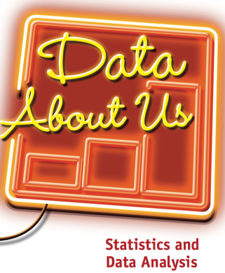6-7 Data About Us

In Data About Us, you will learn different ways to collect, organize, display, and analyze data. In this Unit your student will learn to:

• Use the process of data investigation by posing questions, collecting and analyzing data, and interpreting the data to answer questions
• Organize and represent data using tables, dot plots, line plots, bar graphs, histograms, and box-and-whisker plots
• Describe the overall shape of a distribution and identify whether or not it is symmetrical around a central value
• Compute the mean, median, and mode of a data distribution, and use these measures to indicate what is typical for the distribution
• Describe the variability of a distribution by identifying clusters and gaps, and by calculating the range, Interquartile Range (IQR), and Mean Absolute Deviation (MAD)
• Identify which statistical measures of center and spread should be used to describe a particular distribution of data
• Distinguish between categorical data and numerical data, and identify which graphs and statistics may be used to represent each type of data
• Compare two or more distributions of data, including using measures of center and spread to make comparisons

When you encounter a new problem, it is a good idea to ask yourself questions. In this Unit, you might ask questions such as:

• What question is being investigated to collect these data?
• How might I organize the data?
• What statistical measures will help describe the distribution of data?
• What will these statistical measures tell me about the distribution of the data?
• How can I use graphs and statistics to report an answer to my original question?